#Function Repository Resource:

# RandomScribble

Make a random scribble graphic

Contributed by: Anton Antonov
 ResourceFunction["RandomScribble"][] generates a scribble graphic.

## Details and Options

The scribbles made by ResourceFunction["RandomScribble"] are generated using Bezier curves through random points.
ResourceFunction["RandomScribble"] accepts the same options as Graphics as well as the following options to specify the scribble elements:
 "ColorFunction" Automatic how to colorize the scribble "ConnectingFunction" BezierCurve how to make the scribble strokes "EnvelopeFunctions" None functions for the bottom and top envelopes of the scribble "NumberOfStrokes" 120 number of scribble strokes "OrderedStrokePoints" True whether to order the stroke end points "RotationAngle" Automatic angle used to rotate the scribble PlotStyle {} plot styles used to draw the scribble
There are two modes, single-scribble and multi-scribble. The multi-scribble mode is activated by giving the option "NumberOfStokes" a list of positive integers or by giving the option "RotationAngle" a list of reals.
For the default , in single-scribble mode no colorizing is done and in multi-scribble mode GrayLevel is used.
The function given to ColorFunction is run over Range[0,1,0.1] and the obtained colors are used to colorize the scribble.
Without the use of envelop functions, the random scribbles are generated within the square [-1,1]2.

## Examples

### Basic Examples (3)

Generate a random scribble:

 In:=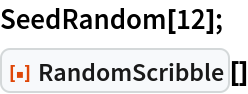Out=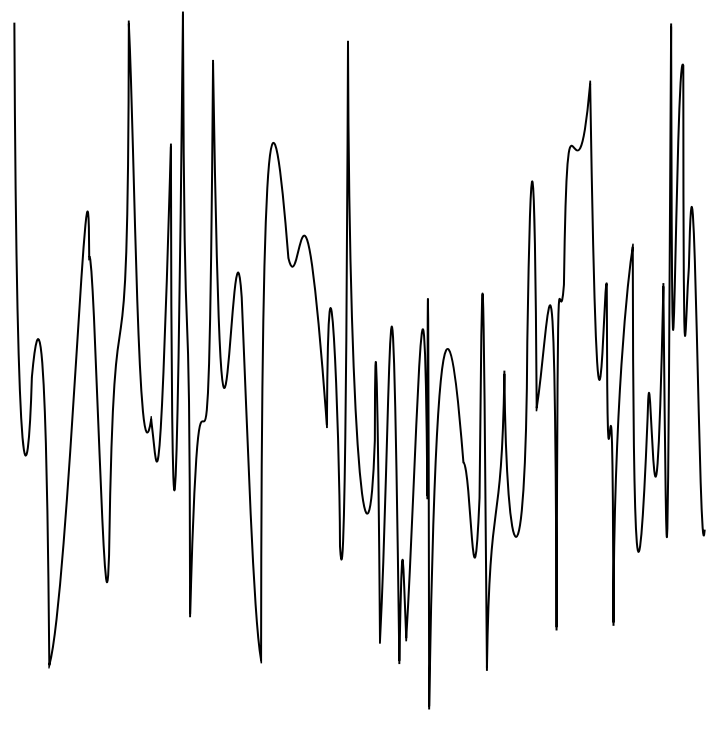Generate a scribble with different option settings:

 In:=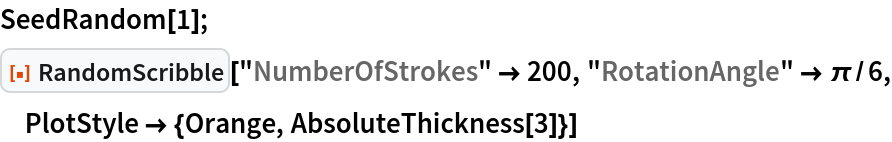Out=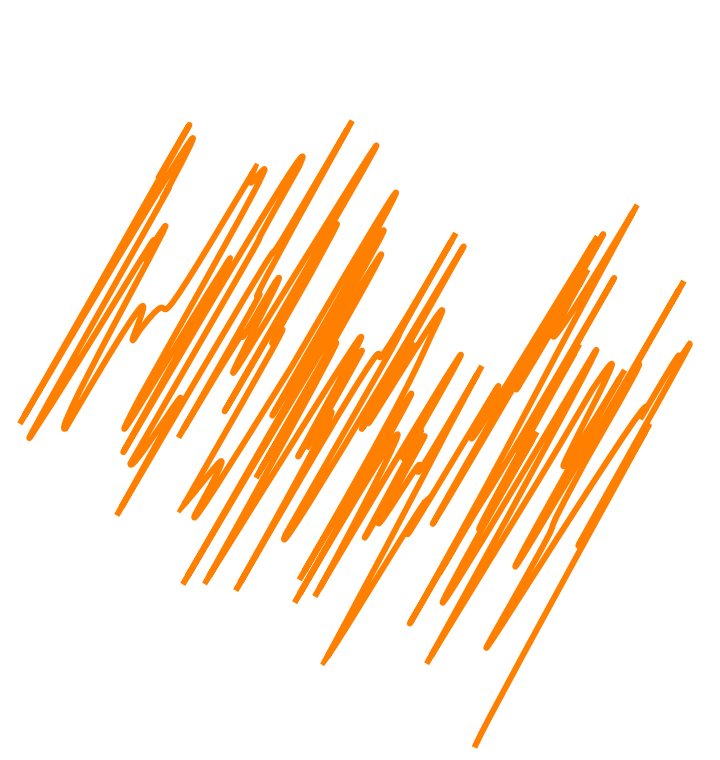Generate a "cross" scribble:

 In:=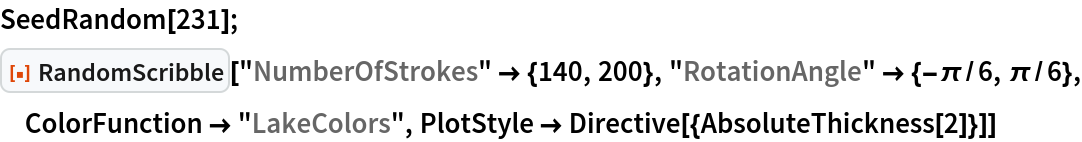Out=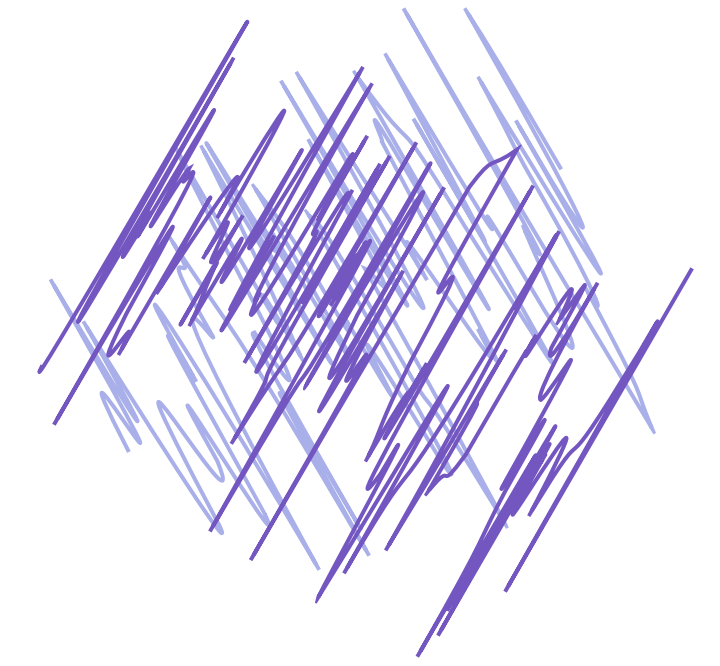### Scope (3)

Here are gray-level and colorized scribbles generated with single-scribble mode:

 In:=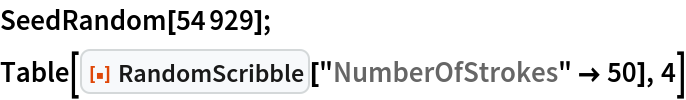Out=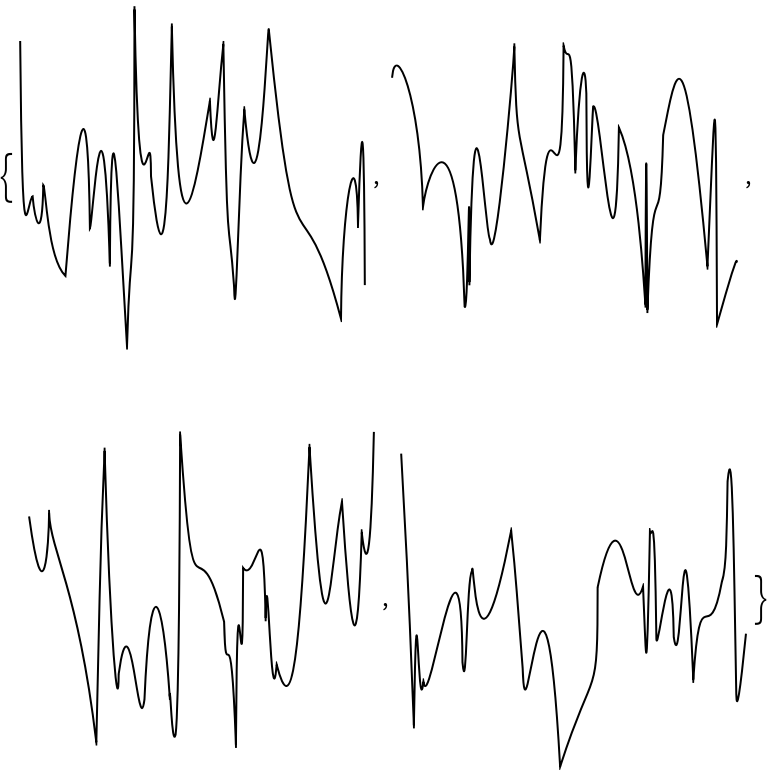In:=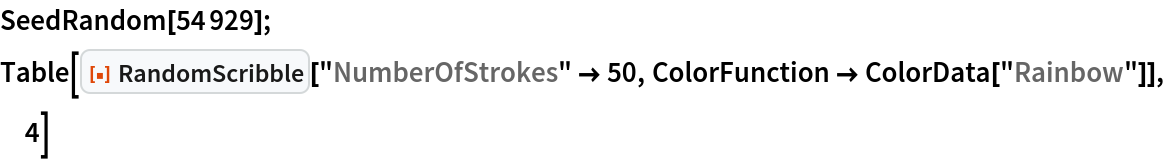Out=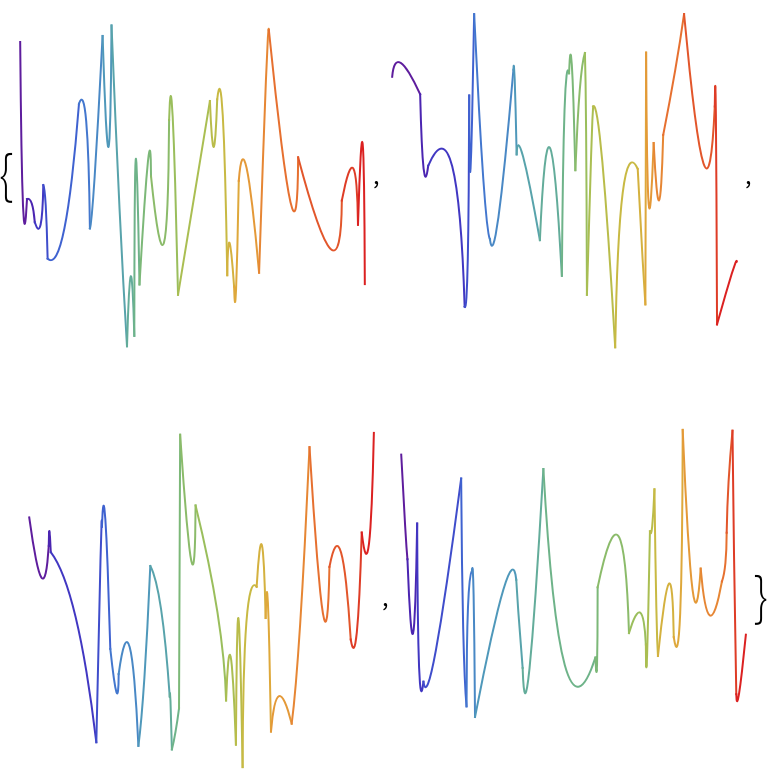Here are gray-level and colorized scribbles generated using multi-scribble mode:

 In:=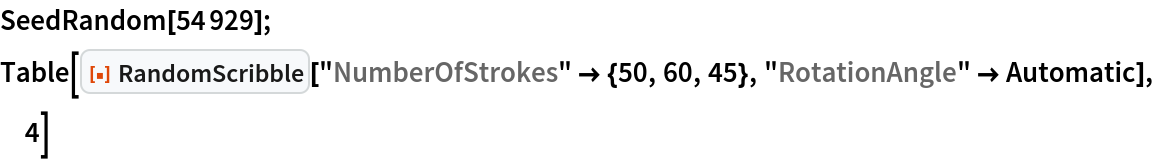Out=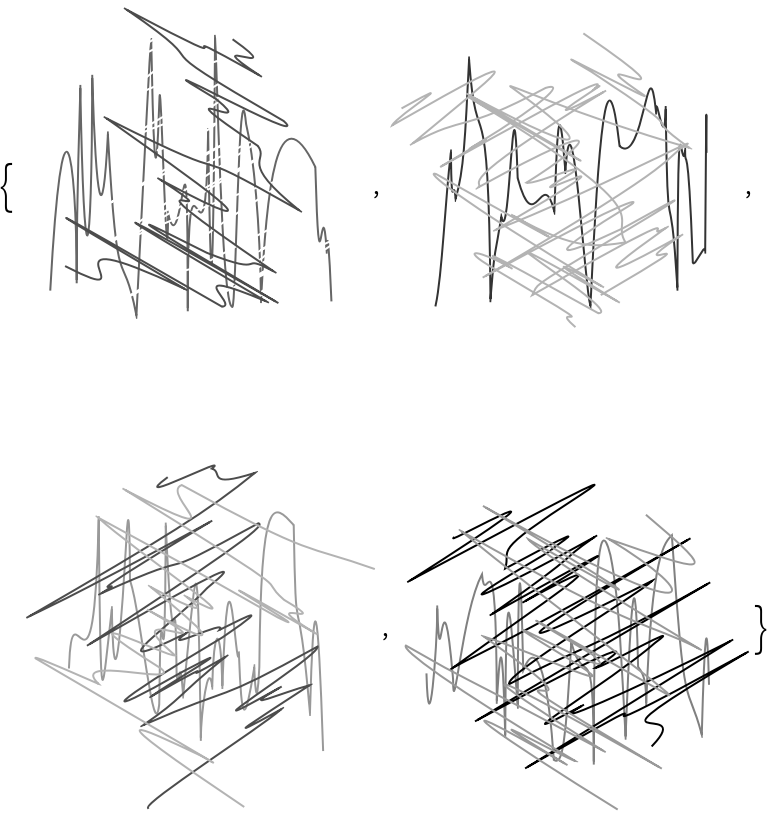In:=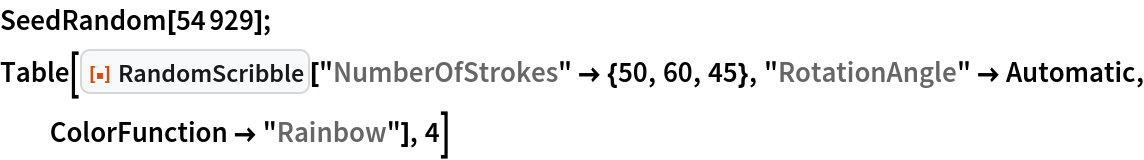Out=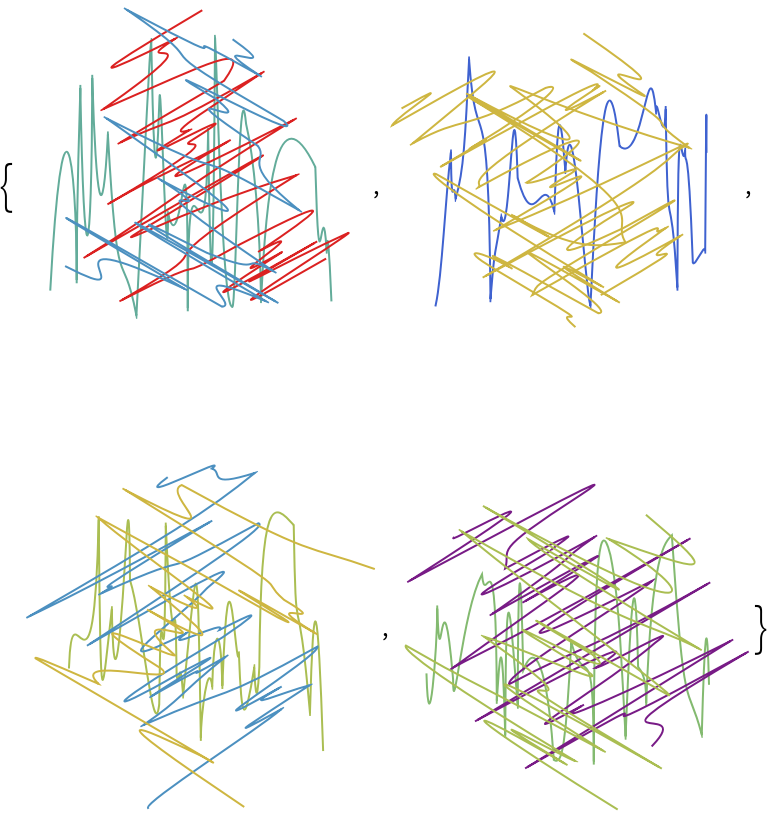Make "orderless" scribbles with fill-ins:

 In:=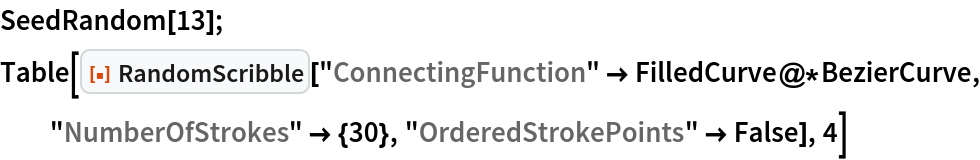Out=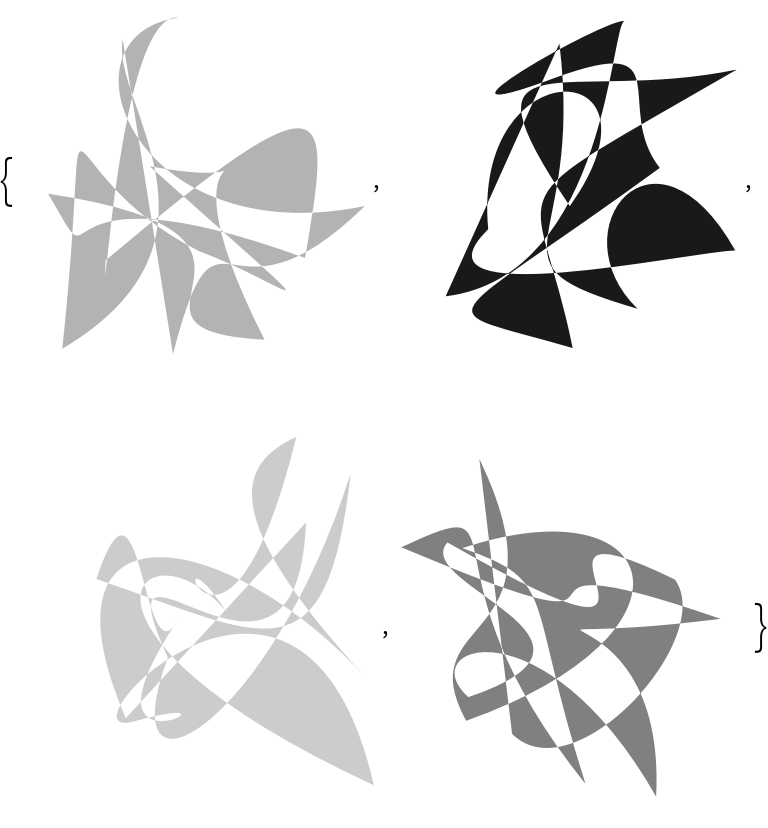### Options (13)

#### ColorFunction (2)

ColorFunction can also take strings that are color schemes known by ColorData.

Here are colorized scribbles generated using single-scribble mode:

 In:=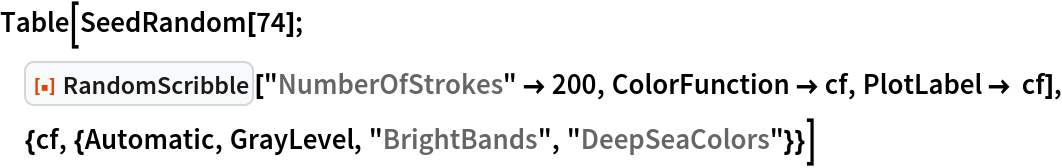Out=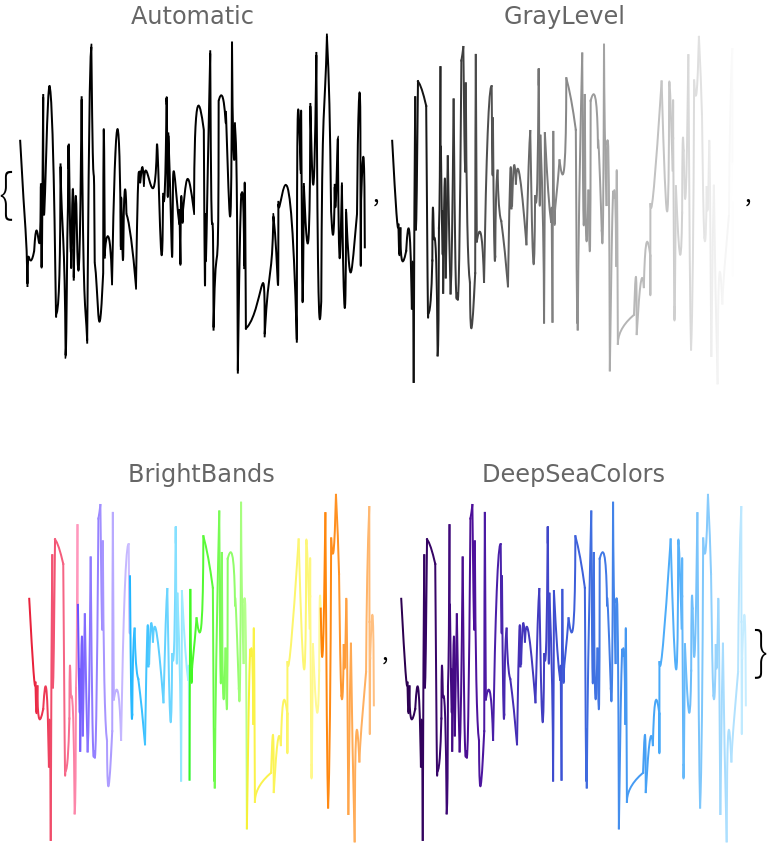Here are scribbles generated using multi-scribble mode:

 In:=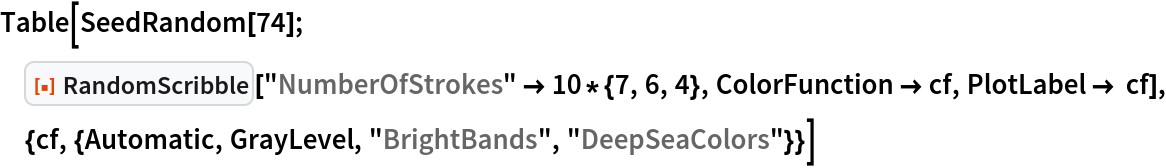Out=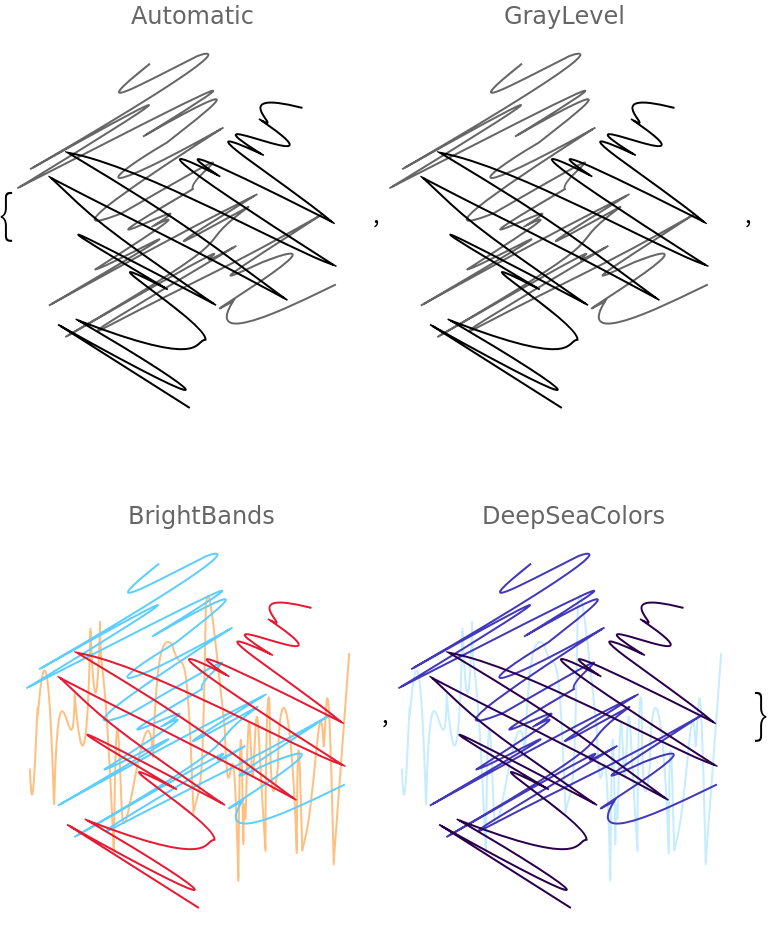#### ConnectingFunction (1)

The option "ConnectingFunction" specifies which graphics primitive to be used between the scribble points:

 In:=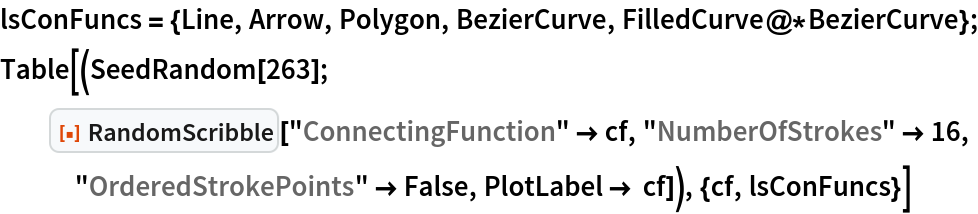Out=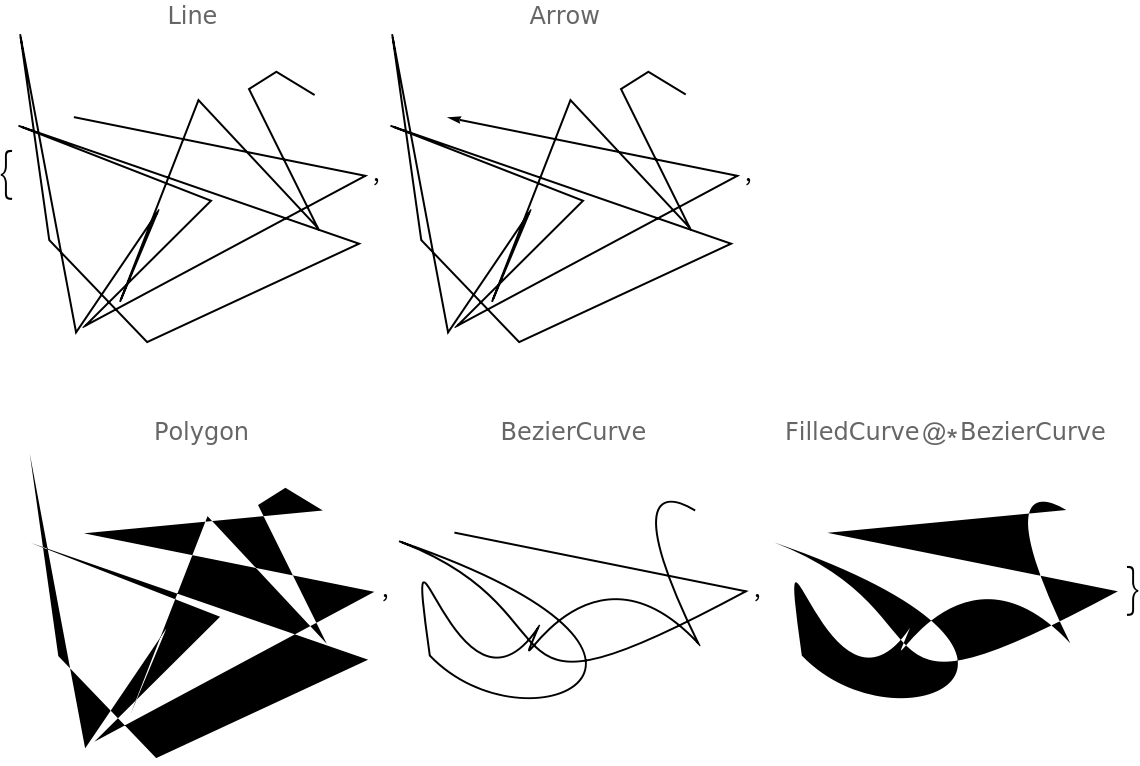#### EnvelopeFunctions (2)

The option "EnvelopeFunctions" specifies functions for the bottom and top envelope curves within which the scribble is drawn. If only one function is given, say F, then that function is considered to be for the top curve and bottom curve function is derived as (-F[#]&):

 In:=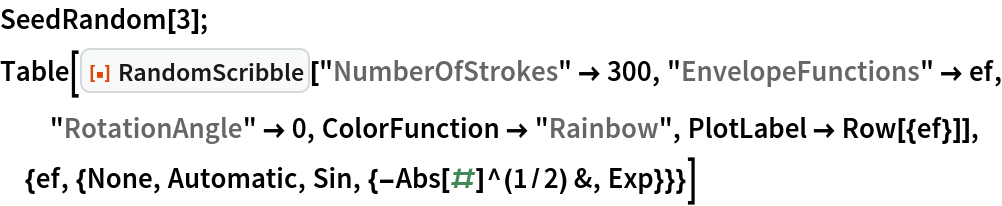Out=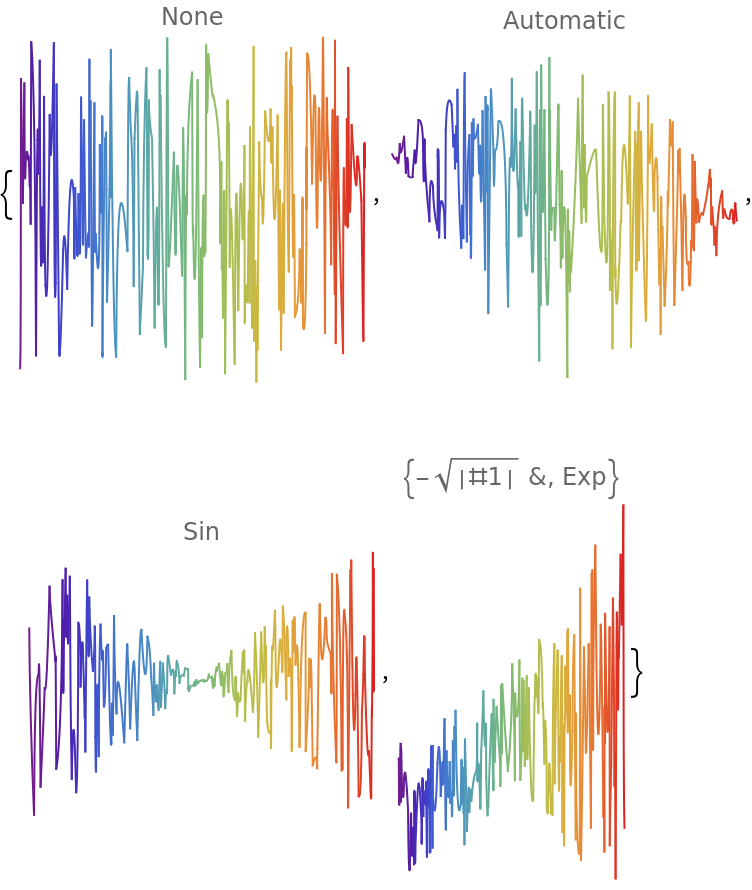If the envelope functions produce complex numbers, the real parts are taken:

 In:=Out=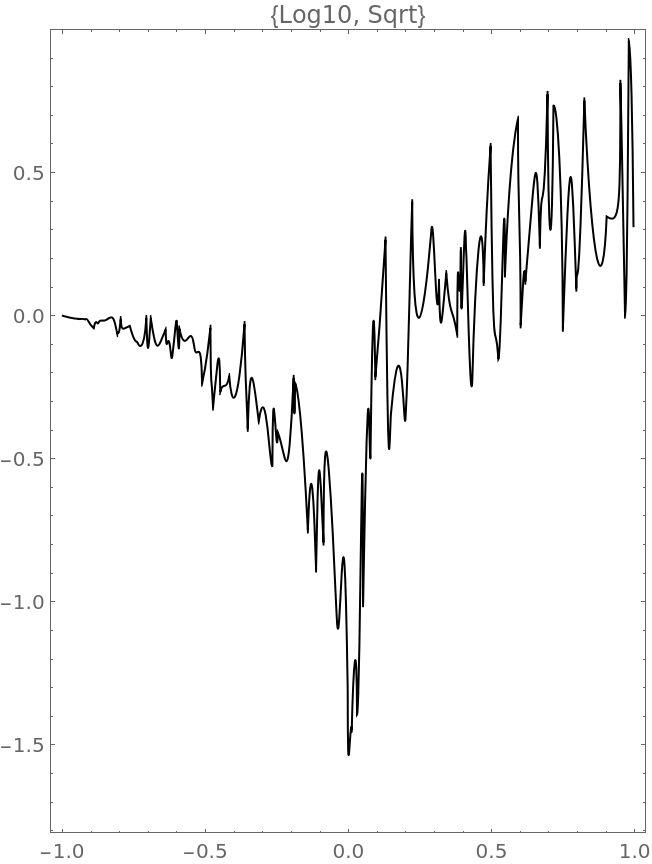#### NumberOfStrokes (2)

The option "NumberOfStrokes" controls how many segments the scribble has:

 In:=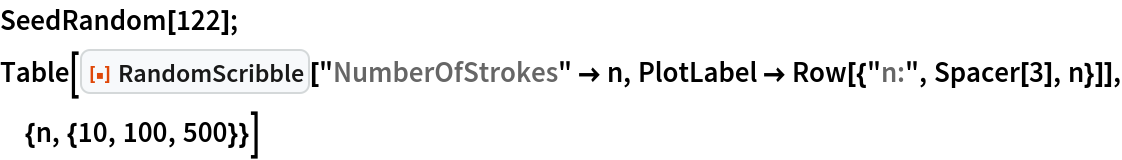Out=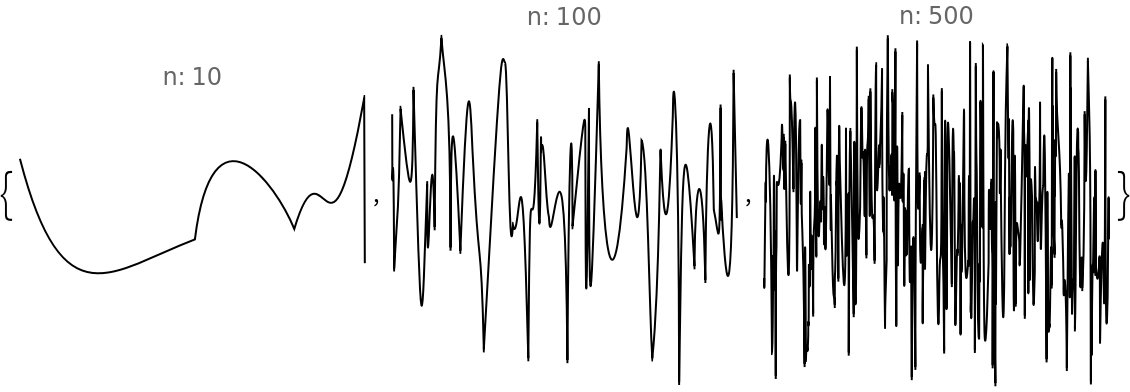If the option "NumberOfStrokes" is given a list of positive integers, then multi-scribble graphics are generated:

 In:=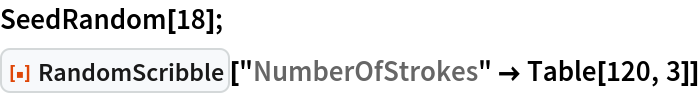Out=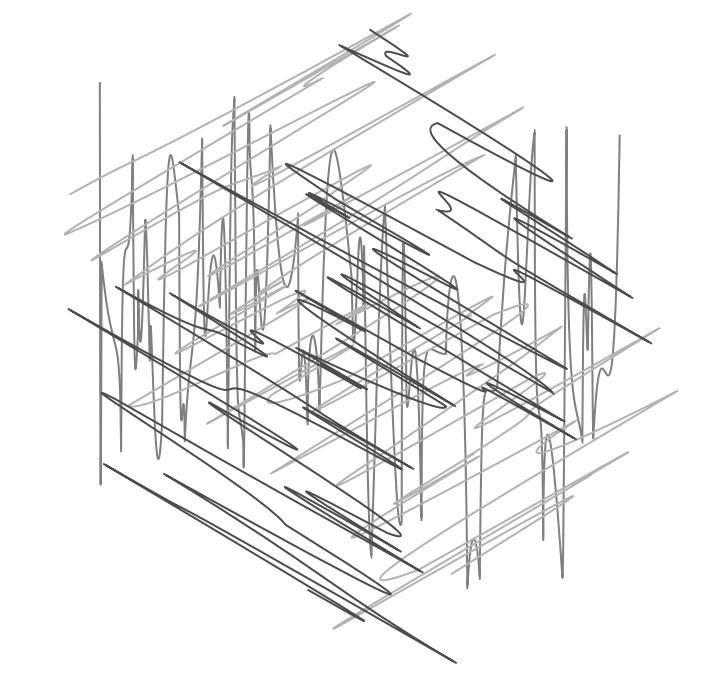#### OrderedStrokePoints (1)

The option "OrderedStrokePoints" controls whether the scribble should be more uniform or completely random:

 In:=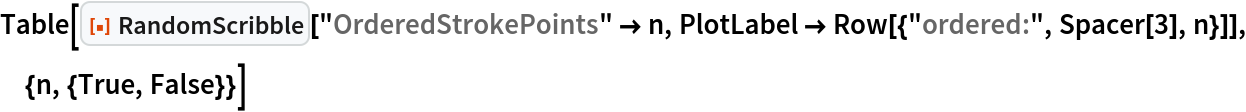Out=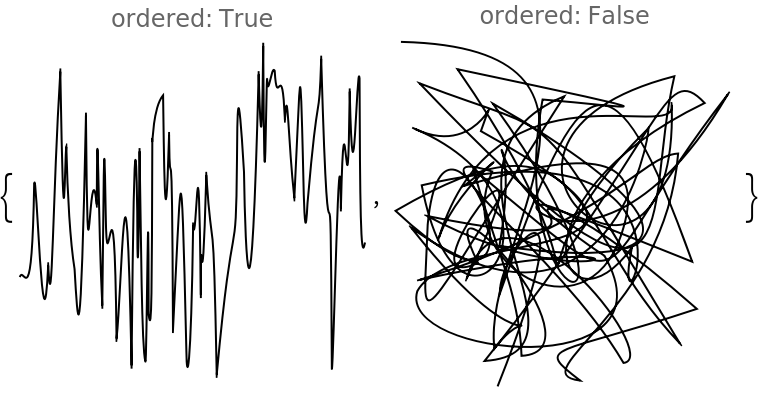#### RotationAngle (3)

The option "RotationAngle" specifies the angle by which the scribble is rotated:

 In:=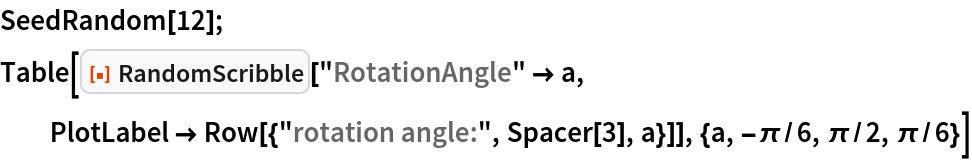Out=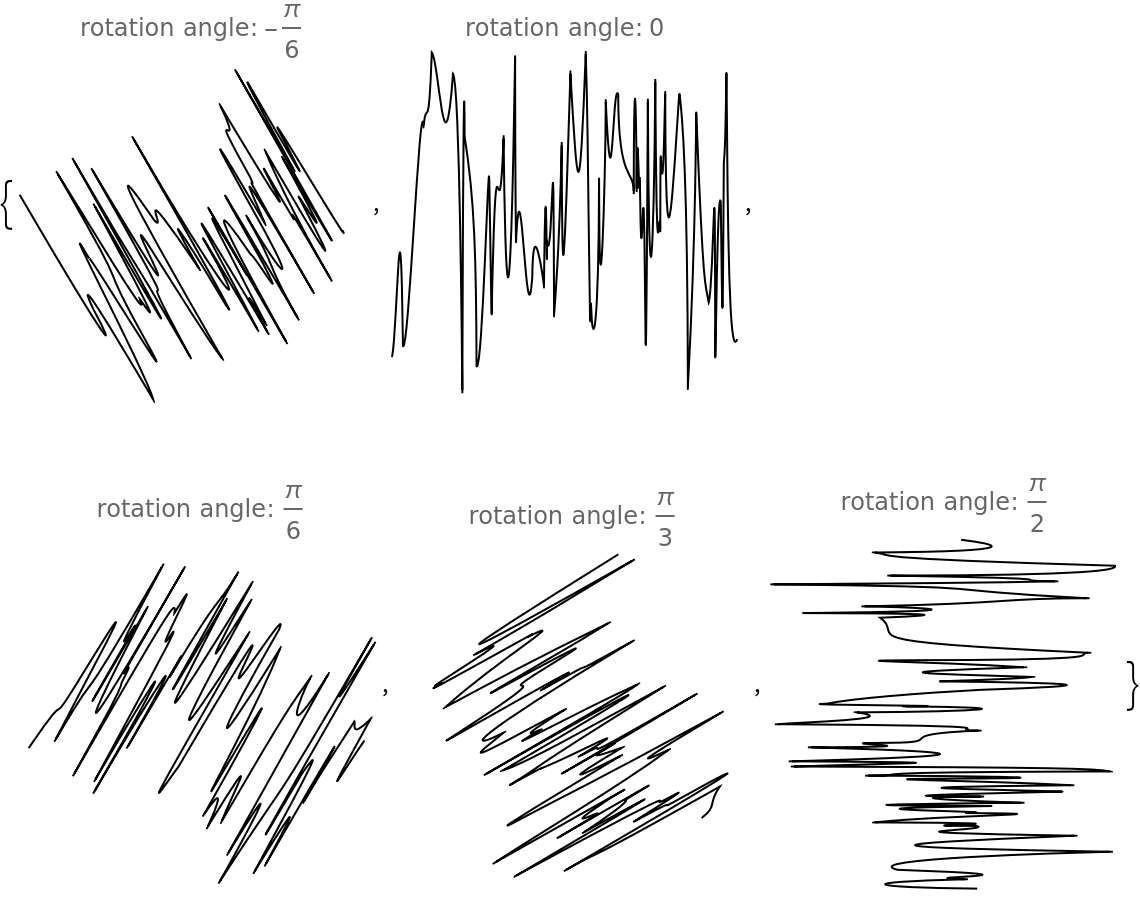If the option "RotationAngle" is given a list of reals, then multi-scribble graphics are generated:

 In:=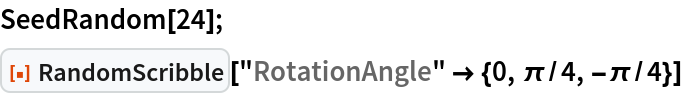Out=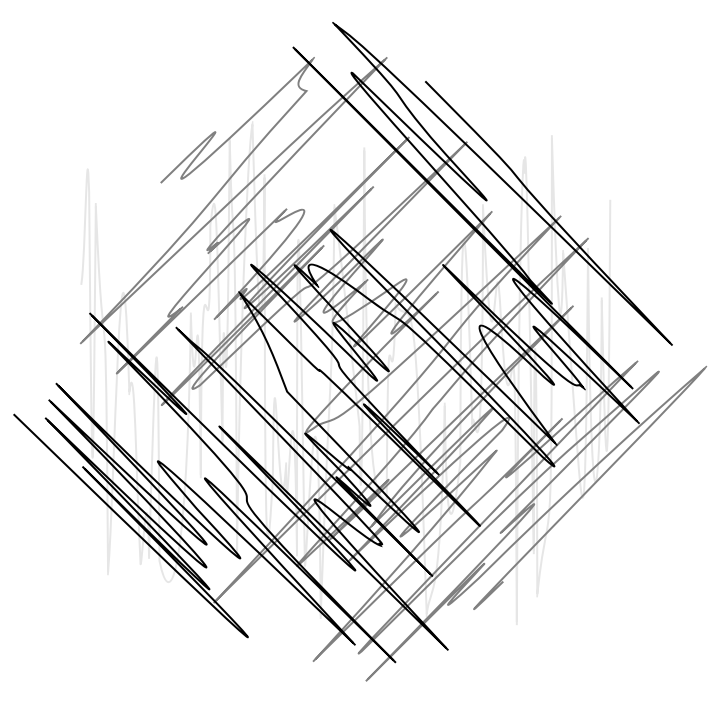In multi-scribble mode, if the value of "RotationAngle" is Automatic, then the generated scribbles are placed over a regular set of rotation angles. If the option value Random is given, then the rotation angles are random:

 In:=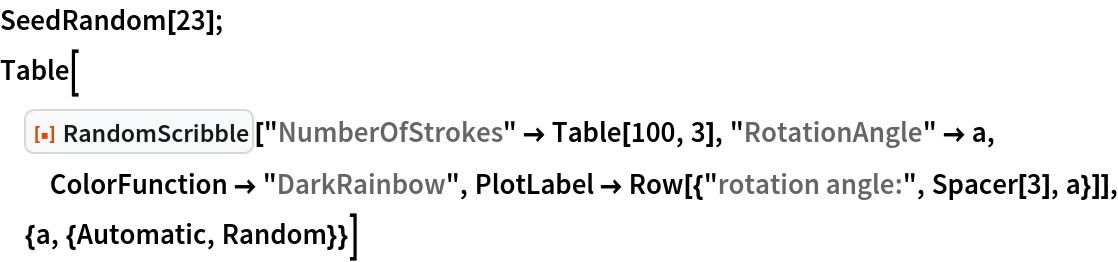Out=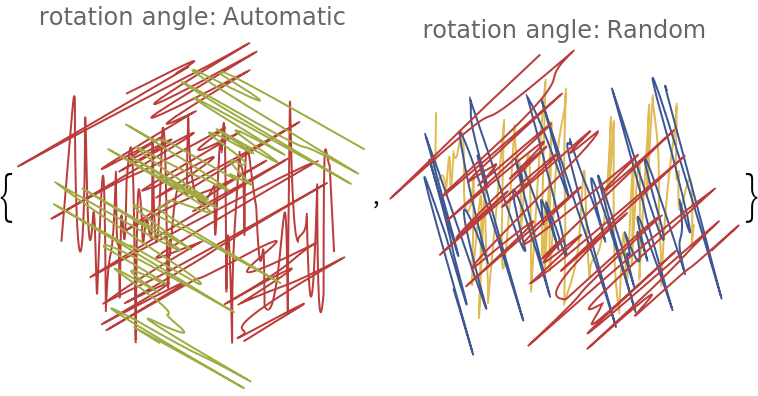#### PlotStyle (2)

With the option PlotStyle, different stylings of the scribble drawing can be specified:

 In:=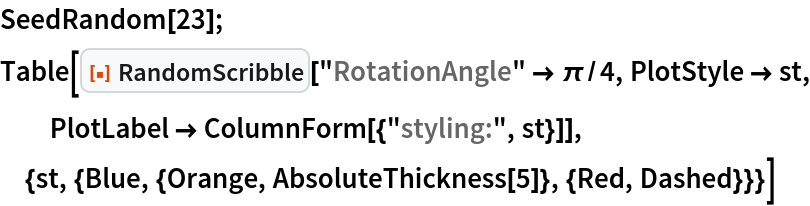Out=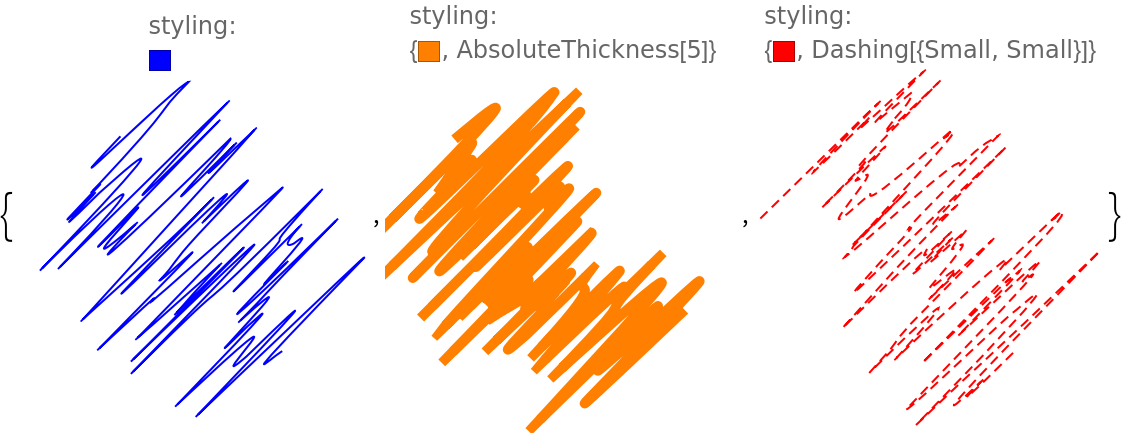In multi-scribble mode, colors specified in PlotStyle are replaced by the colors generated with the option ColorFunction:

 In:=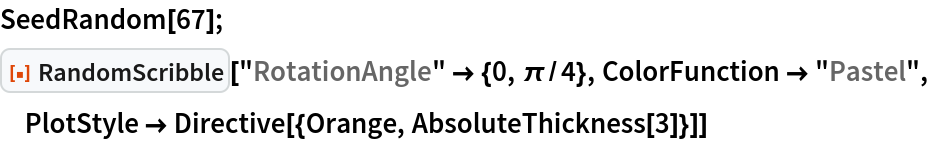Out=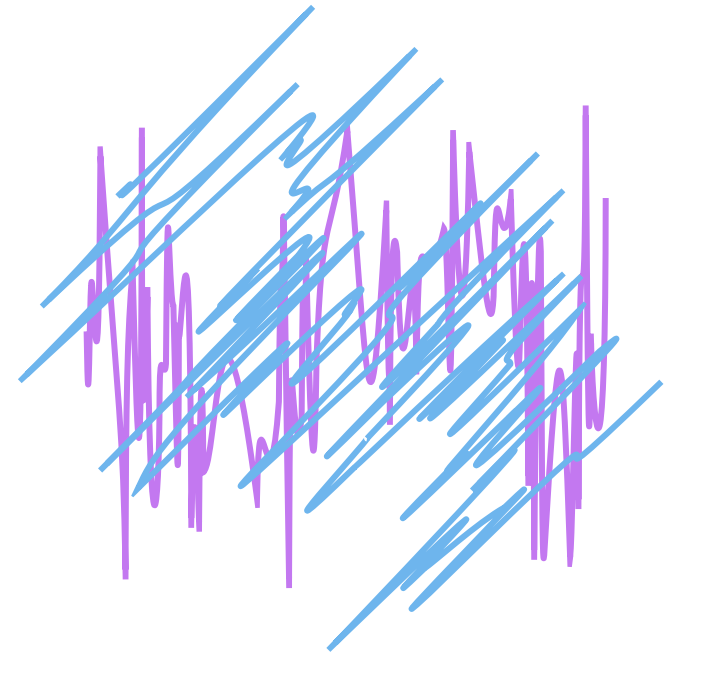### Applications (4)

Combine two colorized single-mode scribbles into a "cross" scribble:

 In:=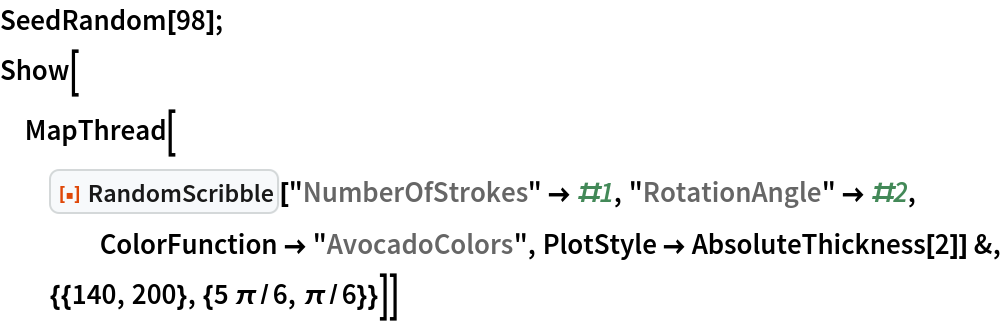Out=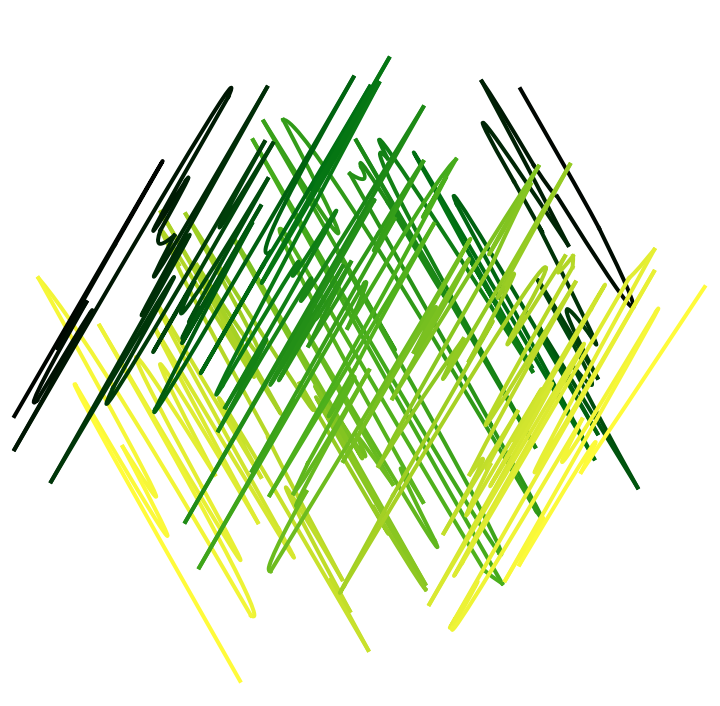Generate a colorized single-mode scribble with a restricted range of a given color scheme and compare it with a scribble that uses the full color scheme range:

 In:=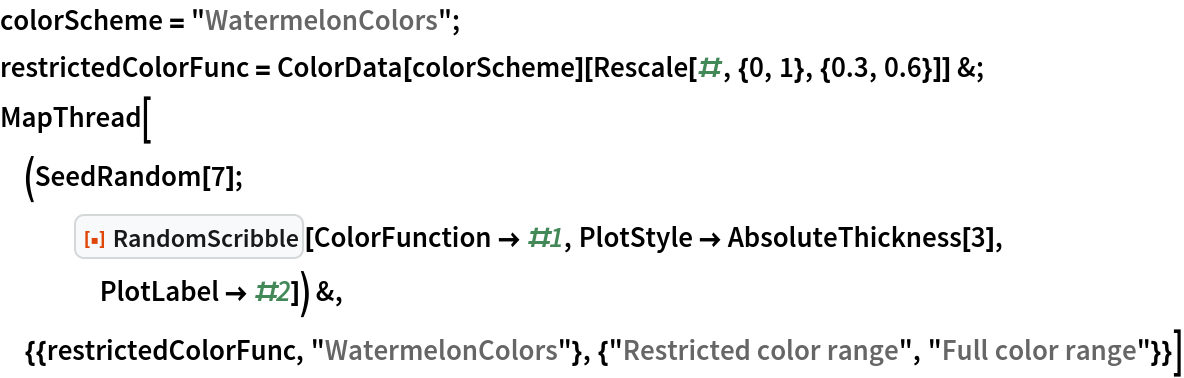Out=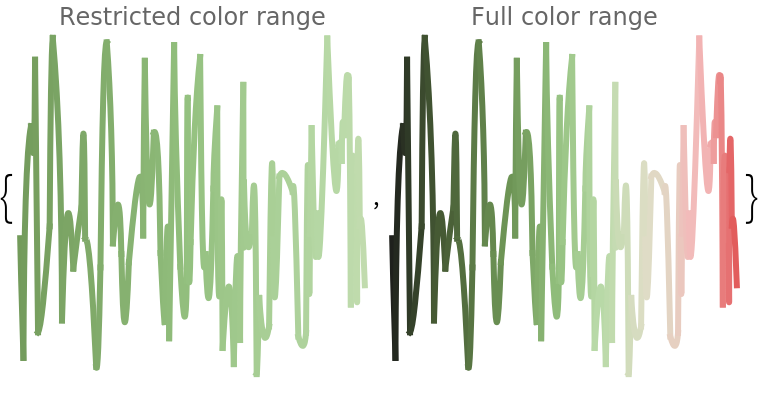Add scribbles to an image:

 In:=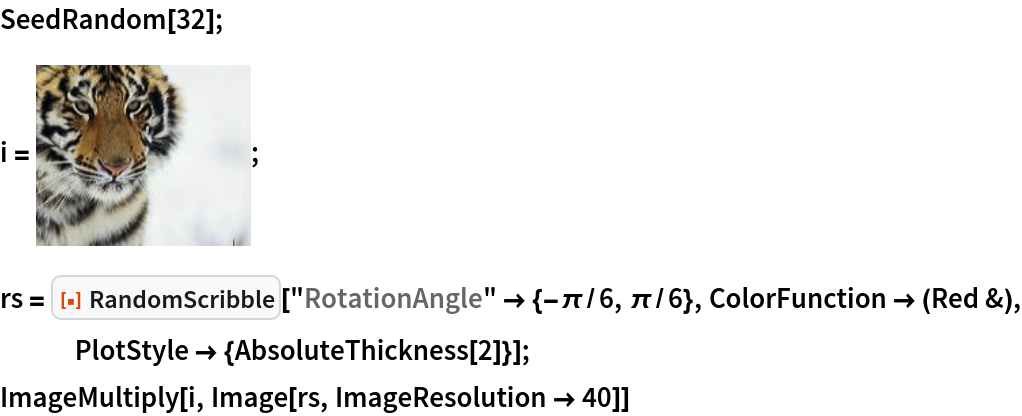Out=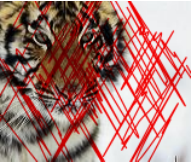Display the elements of a matrix using scribbles:

 In:=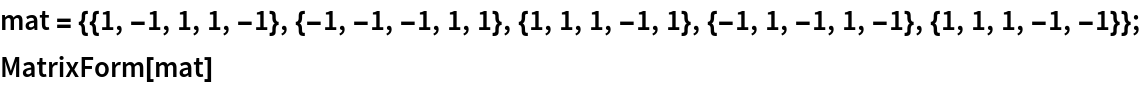Out=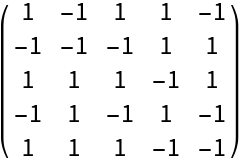Here is the "scribble representation" of the matrix:

 In:=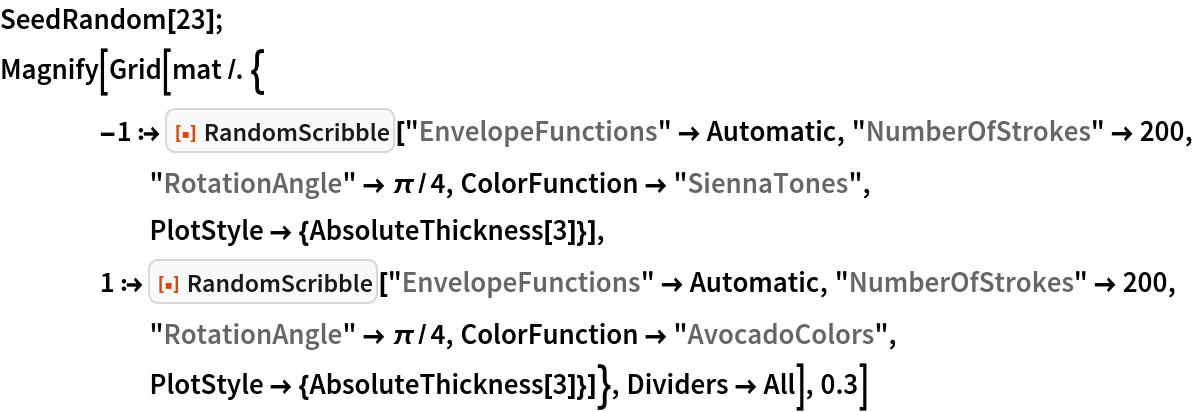Out=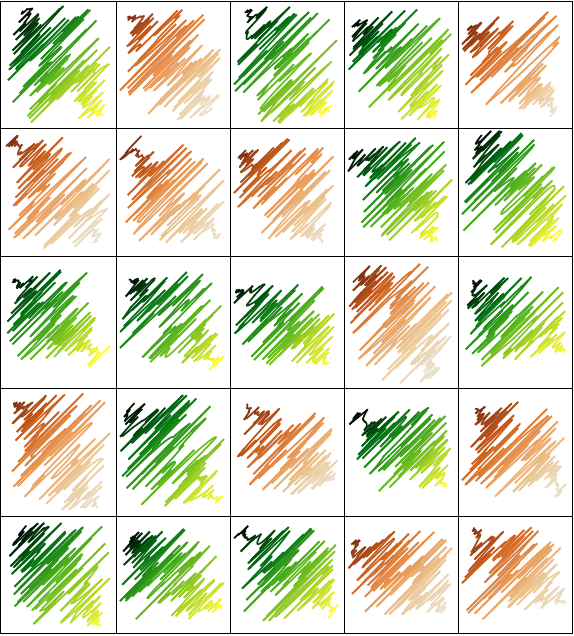### Properties and Relations (2)

Some of the implementation details can be illustrated with appropriate comparison tables. This coloring-versus-connecting table illustrates how the coloring of singe-mode scribbles is implemented:

 In:=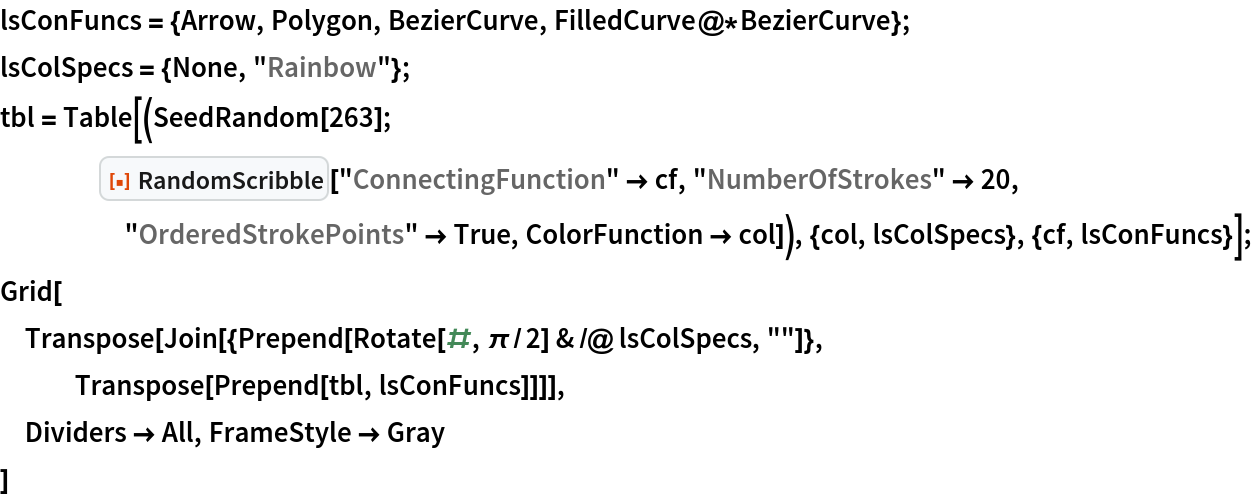Out=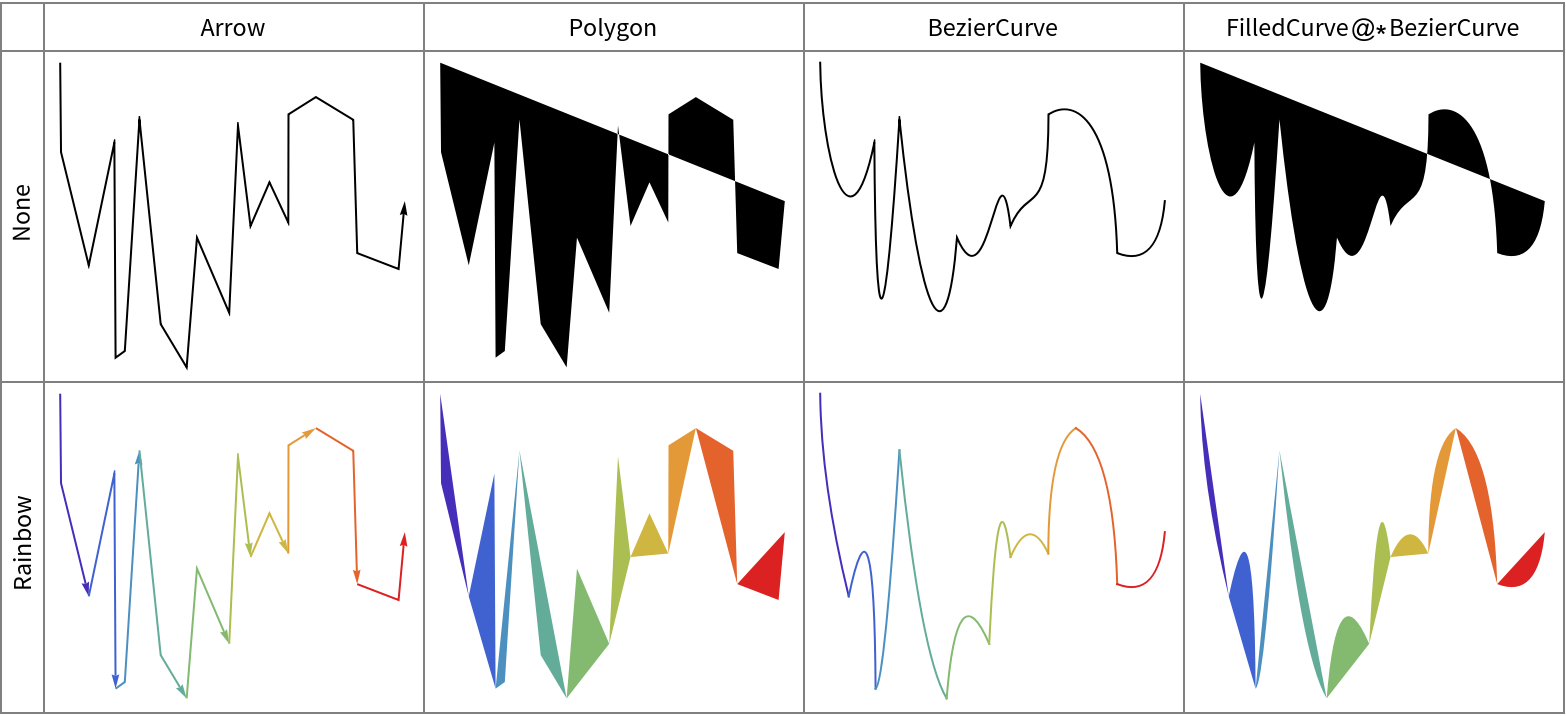RandomScribble can be used in more or less the same way as the resource function RandomMandala. Here, RandomScribble is used with the resource function TexturizePolygons:

 In:=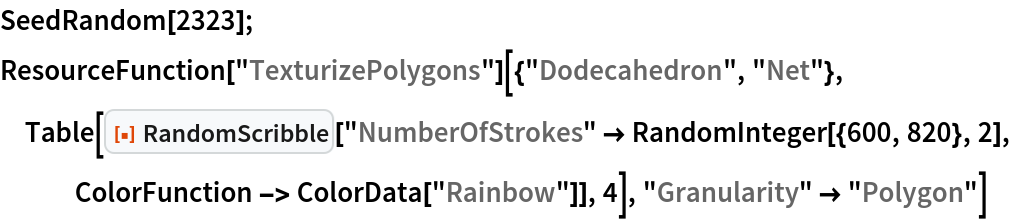Out=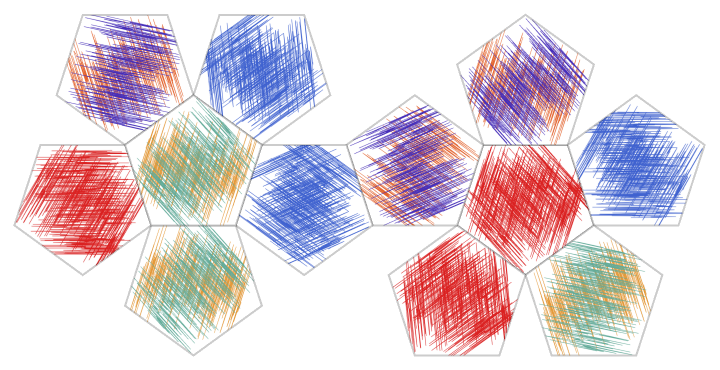Here, the resource function RandomMandala is used with the resource function TexturizePolygons:

 In:=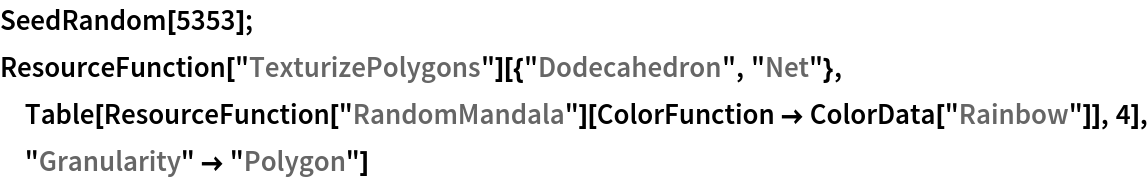Out=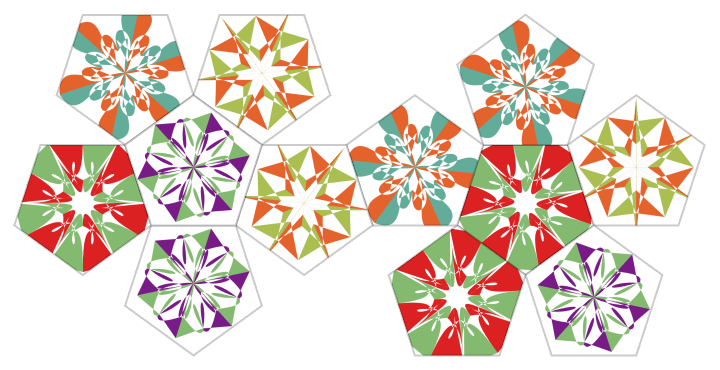### Possible Issues (2)

Specify a constant color function to use the same color for all scribbles in multi-scribble mode:

 In:=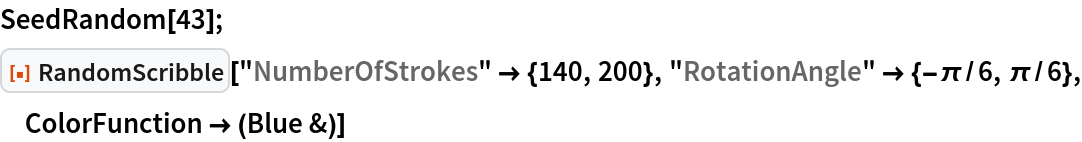Out=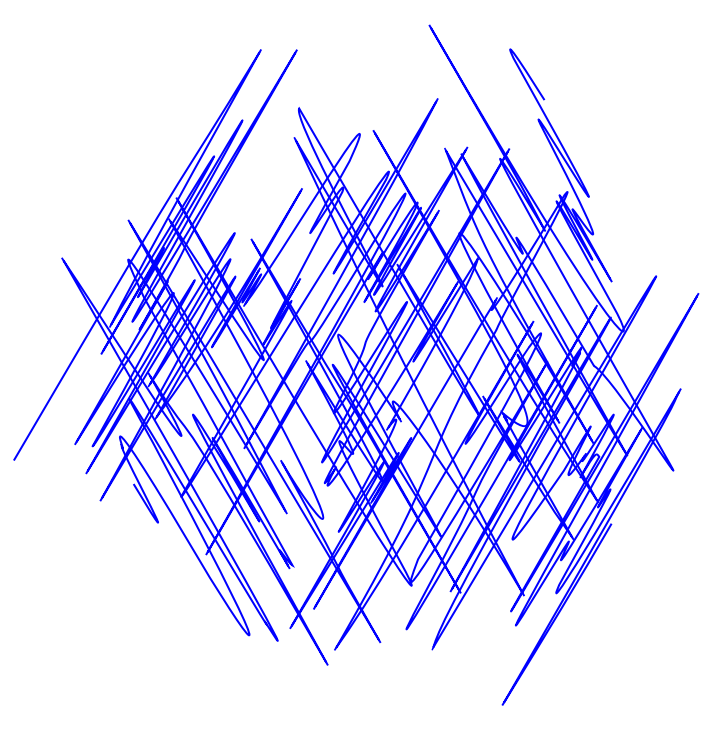Alternatively, specify ColorFunction to be None and PlotStyle to have the desired color:

 In:=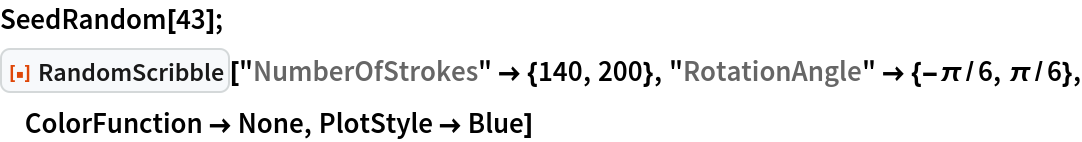Out=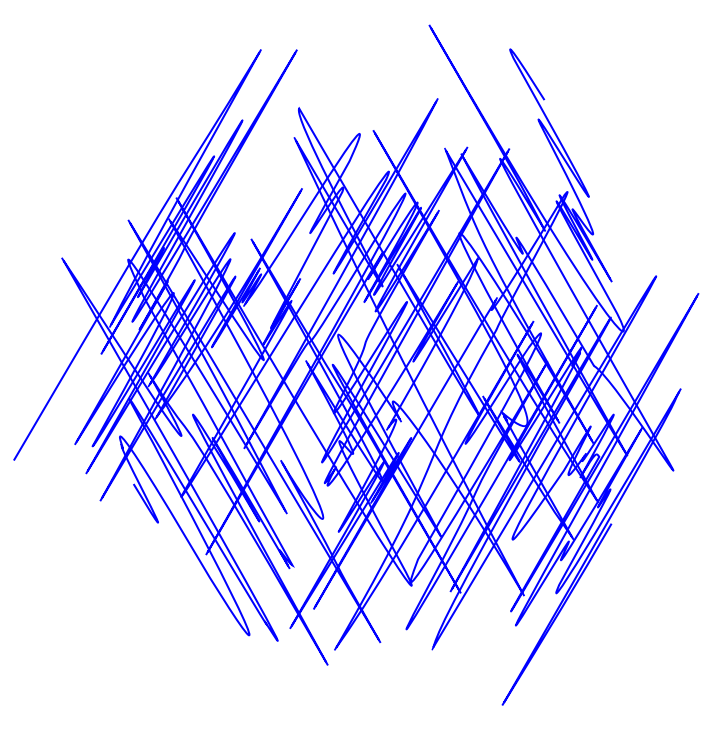### Neat Examples (3)

A table of random scribbles using the "RedBlueTones" color scheme and a regular set of rotation angles:

 In:=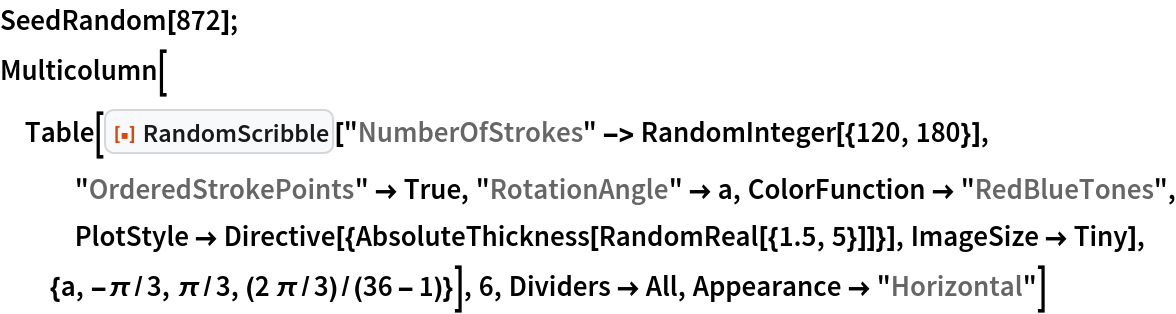Out=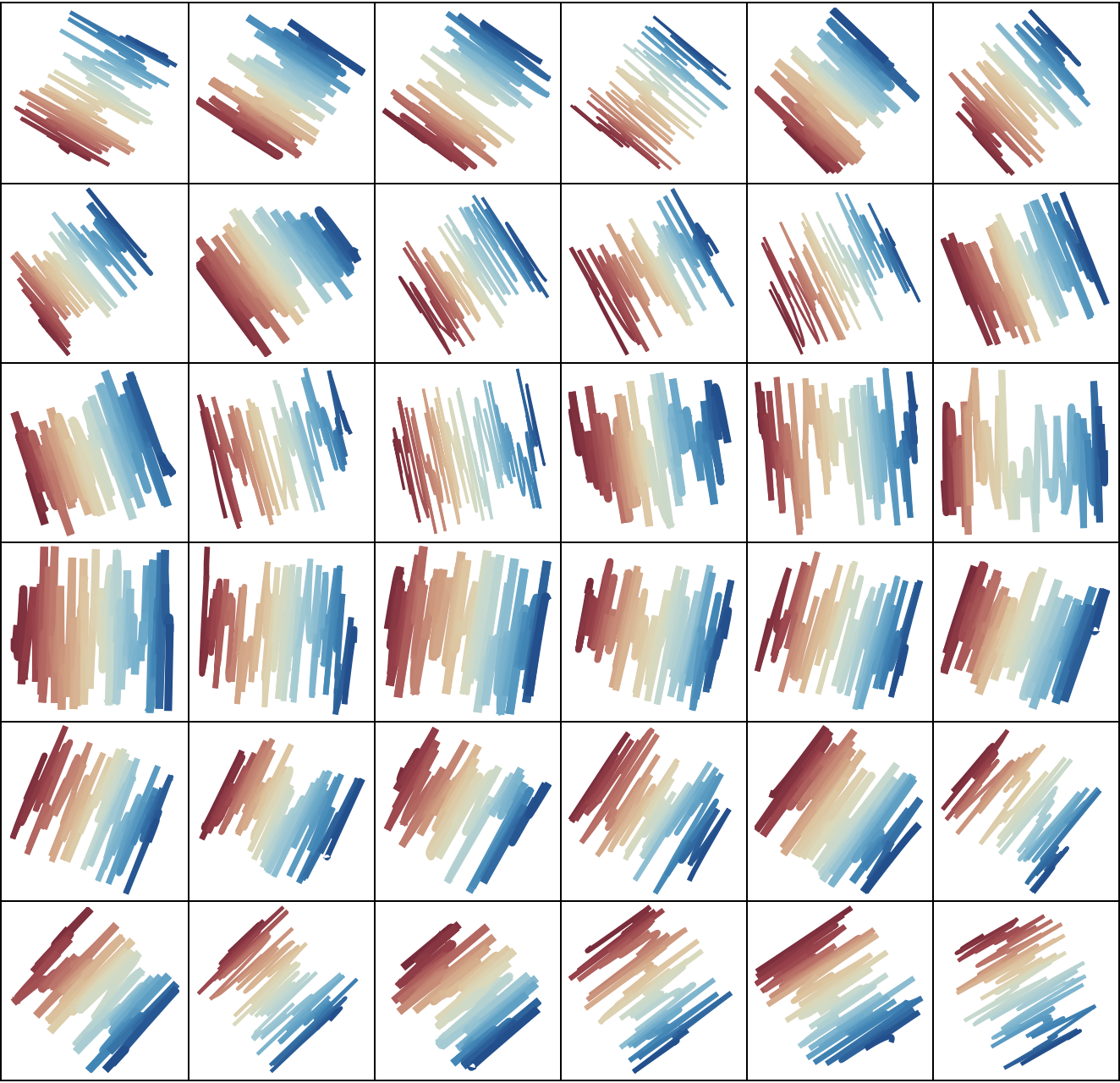A table of random multi-scribble graphics:

 In:=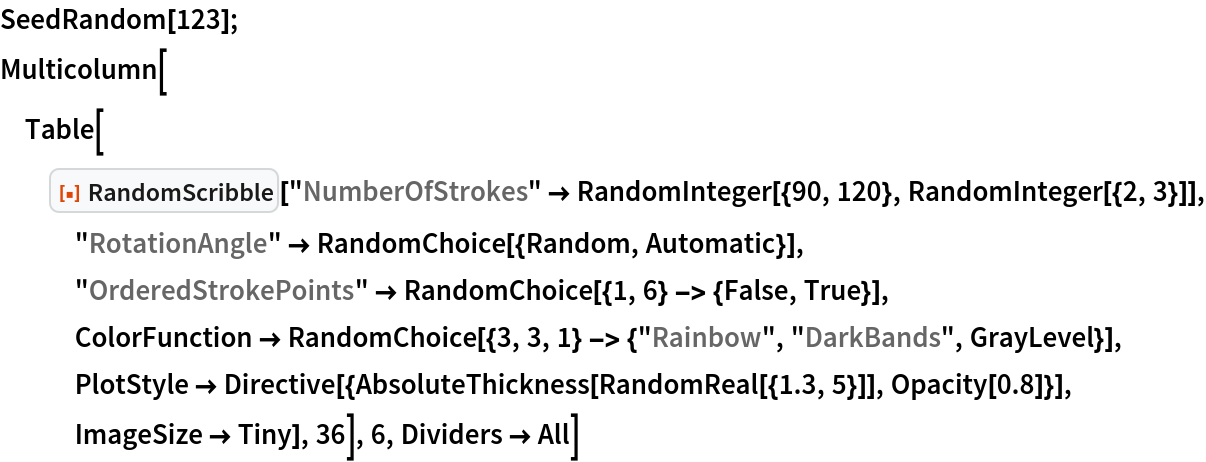Out=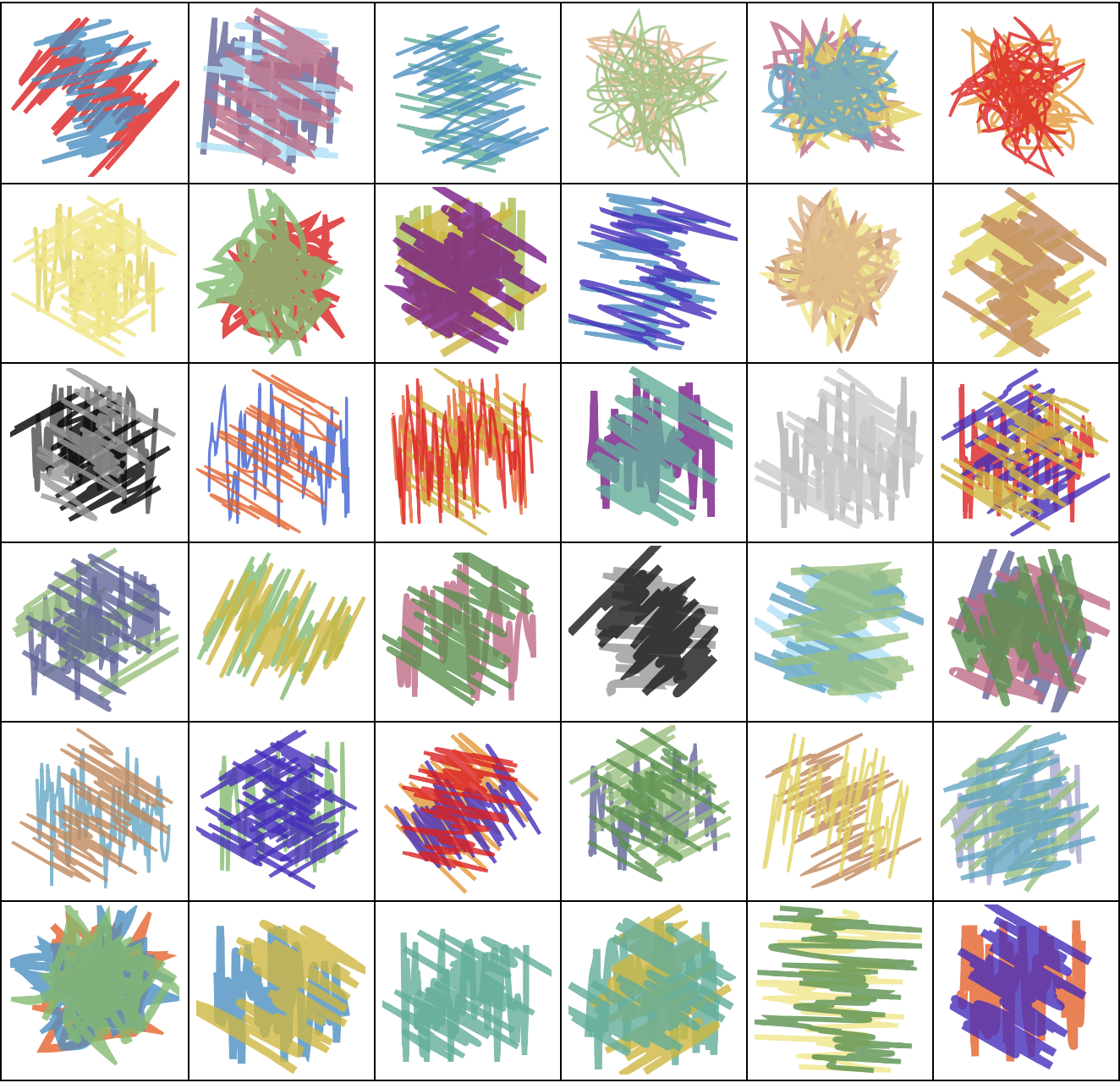A table of random scribbles with unordered stroke points and fill-ins:

 In:=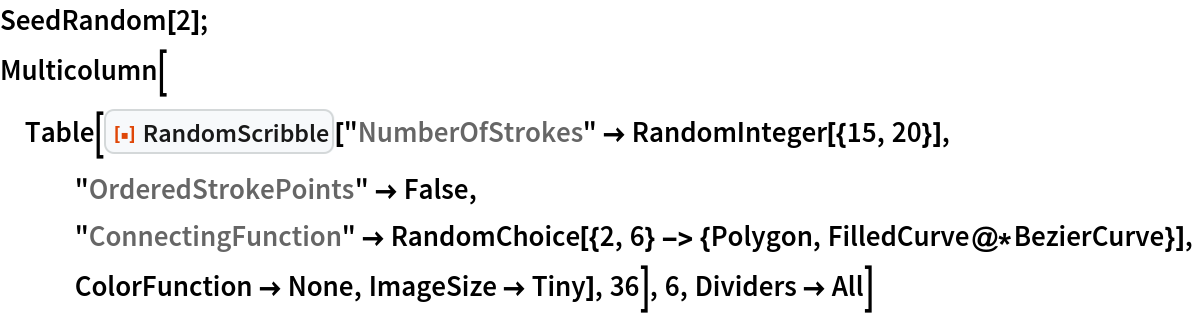Out=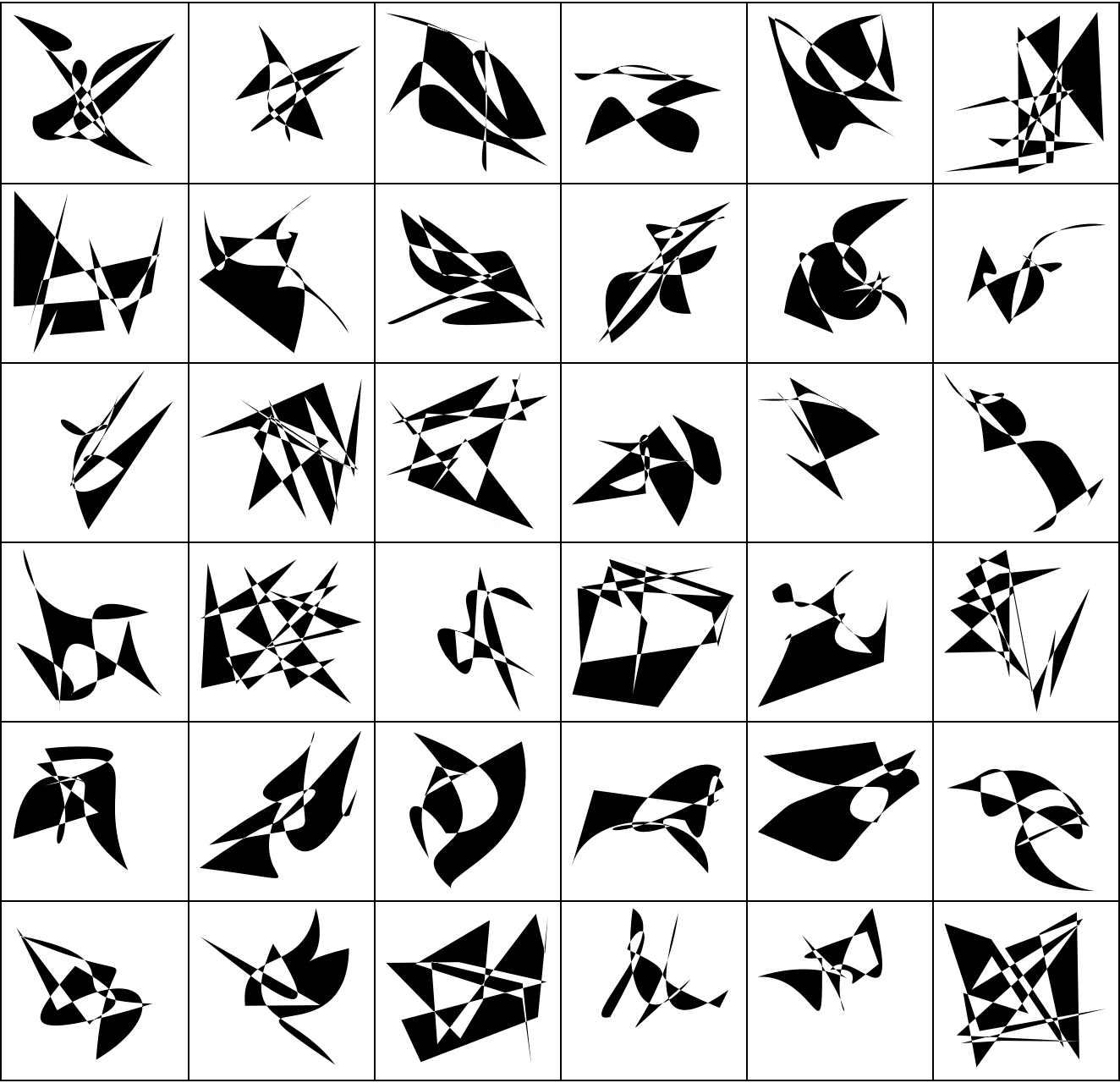Anton Antonov

## Version History

• 2.1.0 – 06 January 2021